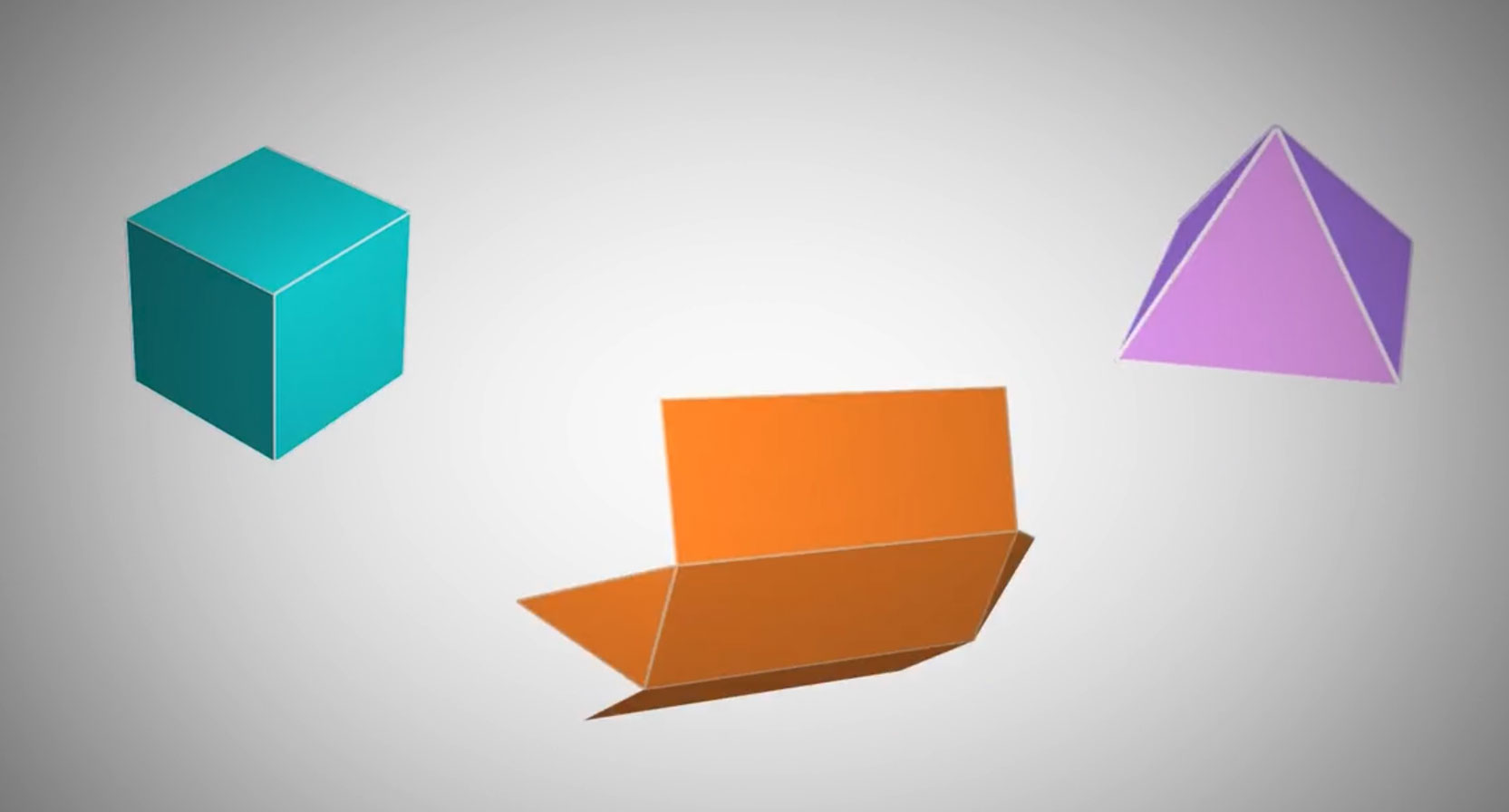# MIDDLE SCHOOL: MATHEMATICS

Curriculum for the Digital Generation

## MIDDLE SCHOOL : MATHEMATICS

Utilizing an innovative math curriculum, students master concepts ranging from data analysis to algebra in an interactive format that is challenging, yet engaging, and exceeds the most demanding academic expectations.

93%

Highly Satisfied

Guaranteed

Satisfaction

Award

Winning

Learner

Centered Design

Outcomes

Based

State Aligned

Designed

## COURSE INTRO VIDEOS

Math 6AMath 6BMath 7AMath 7BMath 8AMath 8B## COURSE CATALOG

#### MATH 6A

In this course, students will build on previously learned concepts like adding, subtracting, multiplying, and dividing.

They will deepen their knowledge of arithmetic with fractions and work with decimals and negative numbers. They will apply these new skills to help solve real-world problems using statistics, ratios, unit conversions, and geometry, as well as expand their ability to write and evaluate expressions, including ones involving new concepts like variables and exponents. Students will also begin working with equations and learn what it means to solve them.

#### MATH 6B

In this course, students will build on previously learned concepts, like positive and negative integers and fractions, to learn about rational numbers and how to compare them.

They will find the distance between points, both on the number line and in the coordinate plane, and then solve geometry problems involving these concepts. They will study the relationships between variables and how to represent them in different ways. They will learn about ratios and unit rates, and then use them to solve real-world problems. Students will also work with data and discover different ways to display data and how to describe data mathematically.

#### MATH 7A

In this course, students begin with adding and multiplying rational numbers by using number lines, rules, and properties.

Then, they move their focus to proportional relationships given in tables, diagrams, graphs, equations, and verbal descriptions. They also learn how to solve problems by finding and comparing unit rates. Next, they rewrite expressions using properties, as well as write and solve simple linear equations by using different methods. The next area of study is probability and statistics, where they will interpret and calculate simple probabilities, as well as learn about populations and samples. Finally, they move on to geometry and learn how to solve problems about scale drawing, circles, and angle relationships, and draw some geometric shapes.

#### MATH 7B

Math 7B helps students level up their skills as a mathematician. The second half of seventh grade math starts by teaching different methods to simplify arithmetic operations, focusing on subtraction and division.

Students learn how to use a variety of strategies such as number lines, rules, and properties. Students then study and interpret proportional relationships, expressions, equations, and inequalities to solve multi-step mathematical and real-life problems.

In the second part of the course, students begin working on compound events. They identify compound events and find their probabilities by using a formula, organized lists, tables, tree diagrams, or simulations. Students then continue with statistics and compare two data sets of random samples by using their center values and variability measures. From there, students make conclusions about the populations the data sets represent. As students near the end of the course, they apply their knowledge to geometry problems. They solve problems that involve area, surface area, volume, two- and three-dimensional objects, and cross sections.

By the end of the course, students should have a solid foundation of the math skills needed to be successful in seventh grade and beyond.

#### MATH 8A

In this course, students begin with the fundamentals of algebra.

They compare, order, and perform operations on rational and irrational numbers; use inverse operations to solve for a variable in one- and two-step equations; write and solve two-step equations from contextual situations; and analyze properties of functions, focusing on linear functions.

The next area of study is very large and very small numbers, where they will solve expressions involving powers of a common base, convert numbers to and from scientific notation, and perform operations on numbers in scientific notation. They will then move on to geometry, where they will perform rigid transformations on figures and prove congruence of figures through a series of rigid transformations.

#### MATH 8B

In this course, students will build on and extend the knowledge they gained in Math 8A.

After reviewing how to solve one- and two-step equations, they are introduced to multi-step equations and proportions. They will apply their knowledge of proportional relationships to geometry, where they perform transformations on figures and prove similarity of figures through a series of rigid transformations and dilations.

Next, students will extend their knowledge of linear relationships by identifying and comparing properties of lines and their equations. Then, students will learn how to solve systems of linear equations using graphs, substitution, and elimination. After that, they build upon their algebraic skills by applying them to statistics, where they analyze and interpret patterns in bivariate data. Finally, students will explore and analyze three-dimensional shapes including cylinders, cones, and spheres.

#### PRE-ALGEBRA

Pre-Algebra is designed to give students the opportunity to build the conceptual understanding and skills necessary to be successful in Algebra 1A and Algebra 1B.

Students begin this course by reviewing operations with rational numbers. They find factors and multiples of numbers, along with common factors and multiples of sets of numbers. They also add, subtract, multiply, and divide integers, fractions, and mixed numbers. Students apply properties, such as the Distributive, Associative, and Commutative Properties, and use the order of operations to simplify numerical expressions and then algebraic expressions. Next, they work with algebraic equations and use them to solve problems. This is followed by identifying, graphing, and comparing linear relationships. The course concludes with an introduction to functions.